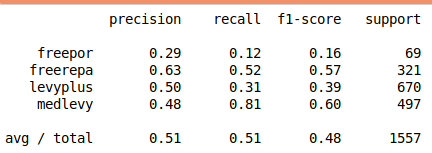# Naive Bayes with Python

Naive Bayes is a probabilistic classifier that is often employed when you have multiple or more than two classes in which you want to place your data. This algorithm is particularly used when you dealing with text classification with large datasets and many features.If you are more familiar with statistics you know that Bayes developed a method of probability that is highly influential today. In short, his system takes into conditional probability. In the case of naive Bayes,  the classifier assumes that the presence of a certain feature in a class is not related to the presence of any other feature. This assumption is why Naive Bayes is Naive.

For our purposes, we will use Naive Bayes to predict the type of insurance a person has in the DoctorAUS dataset in the pydataset module. Below is some initial code.

```from pydataset import data
import pandas as pd
from sklearn.naive_bayes import GaussianNB
from sklearn.model_selection import train_test_split
from sklearn.metrics import classification_report```

Next, we will load our dataset DoctorAUS. Then we will separate the independent variables that we will use from the dependent variable of insurance in two different datasets. If you want to know more about the dataset and the variables you can type data(“DoctorAUS”, show_doc=True)

```df=data("DoctorAUS")
y=df['insurance']```

Now, we will create our train and test datasets. We will do a 70/30 split. We will also use Gaussian Naive Bayes as our algorithm. This algorithm assumes the data is normally distributed. There are other algorithms available for Naive Bayes as well.  We will also create our model with the .fit function.

```X_train,X_test,y_train,y_test=train_test_split(X,y,test_size=.3,random_state=0)
clf=GaussianNB()
clf.fit(X_train,y_train)```

Finally, we will predict with our model and run the classification report to determine the success of the model.

```y_pred=clf.predict(X_test)
print(classification_report(y_test,y_pred))```You can see that our overall numbers are not that great. This means that the current algorithm is probably not the best choice for classification. Of course, there could other problems as well that need to be explored.

Conclusion

This post was simply a demonstration of how to conduct an analysis with Naive Bayes using Python. The process is not all that complicate and is similar to other algorithms that are used.